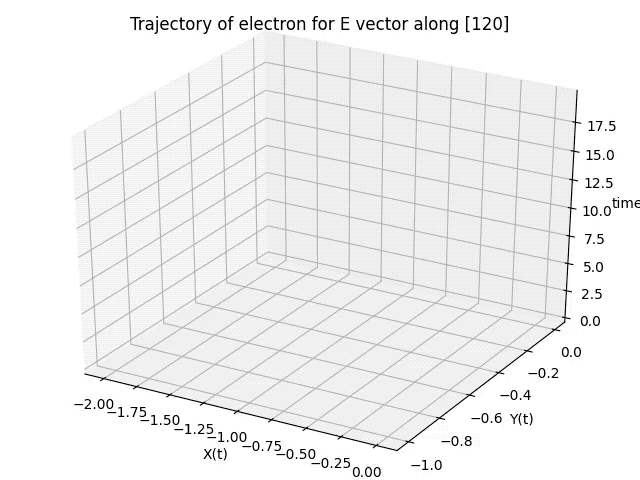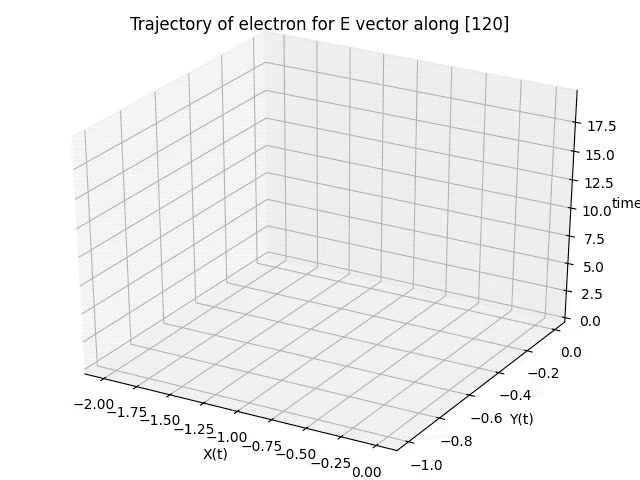# 3D Trajectory Animated using Matplotlib (Python)

Based on a lot of useful references especially (https://gist.github.com/neale/e32b1f16a43bfdc0608f45a504df5a84) here I share my code to plot the animated trajectory of a particle (in this case electron) using a 3D line/scatter chart.

In my last two blog posts I have already shown how to create an animated 2D line/scatter plot and how to plot a 3D chart.
Here we combine the two codes.

If you just want a line chart then use the following:

### CODE

```import numpy as np
import matplotlib.pyplot as plt
import matplotlib.animation as animation
from mpl_toolkits.mplot3d import Axes3D

# References
# https://gist.github.com/neale/e32b1f16a43bfdc0608f45a504df5a84
# https://towardsdatascience.com/animations-with-matplotlib-d96375c5442c
# https://riptutorial.com/matplotlib/example/23558/basic-animation-with-funcanimation

# ANIMATION FUNCTION
def func(num, dataSet, line):
# NOTE: there is no .set_data() for 3 dim data...
line.set_data(dataSet[0:2, :num])
line.set_3d_properties(dataSet[2, :num])
return line

# THE DATA POINTS
t = np.arange(0,20,0.2) # This would be the z-axis ('t' means time here)
x = np.cos(t)-1
y = 1/2*(np.cos(2*t)-1)
dataSet = np.array([x, y, t])
numDataPoints = len(t)

# GET SOME MATPLOTLIB OBJECTS
fig = plt.figure()
ax = Axes3D(fig)

# NOTE: Can't pass empty arrays into 3d version of plot()
line = plt.plot(dataSet, dataSet, dataSet, lw=2, c='g') # For line plot

# AXES PROPERTIES]
# ax.set_xlim3d([limit0, limit1])
ax.set_xlabel('X(t)')
ax.set_ylabel('Y(t)')
ax.set_zlabel('time')
ax.set_title('Trajectory of electron for E vector along ')

# Creating the Animation object
line_ani = animation.FuncAnimation(fig, func, frames=numDataPoints, fargs=(dataSet,line), interval=50, blit=False)
#line_ani.save(r'AnimationNew.mp4')

plt.show()

```

### OUTPUTFor a combination of Scatter and Line chart use the following code

### CODE

```import numpy as np
import matplotlib.pyplot as plt
import matplotlib.animation as animation
from mpl_toolkits.mplot3d import Axes3D

# References
# https://gist.github.com/neale/e32b1f16a43bfdc0608f45a504df5a84
# https://towardsdatascience.com/animations-with-matplotlib-d96375c5442c
# https://riptutorial.com/matplotlib/example/23558/basic-animation-with-funcanimation

# ANIMATION FUNCTION
def func(num, dataSet, line, redDots):
# NOTE: there is no .set_data() for 3 dim data...
line.set_data(dataSet[0:2, :num])
line.set_3d_properties(dataSet[2, :num])
redDots.set_data(dataSet[0:2, :num])
redDots.set_3d_properties(dataSet[2, :num])
return line

# THE DATA POINTS
t = np.arange(0,20,0.2) # This would be the z-axis ('t' means time here)
x = np.cos(t)-1
y = 1/2*(np.cos(2*t)-1)
dataSet = np.array([x, y, t])
numDataPoints = len(t)

# GET SOME MATPLOTLIB OBJECTS
fig = plt.figure()
ax = Axes3D(fig)
redDots = plt.plot(dataSet, dataSet, dataSet, lw=2, c='r', marker='o') # For scatter plot
# NOTE: Can't pass empty arrays into 3d version of plot()
line = plt.plot(dataSet, dataSet, dataSet, lw=2, c='g') # For line plot

# AXES PROPERTIES]
# ax.set_xlim3d([limit0, limit1])
ax.set_xlabel('X(t)')
ax.set_ylabel('Y(t)')
ax.set_zlabel('time')
ax.set_title('Trajectory of electron for E vector along ')

# Creating the Animation object
line_ani = animation.FuncAnimation(fig, func, frames=numDataPoints, fargs=(dataSet,line,redDots), interval=50, blit=False)
# line_ani.save(r'Animation.mp4')

plt.show()
```

### OUTPUT[wpedon id="7041" align="center"]

## 2 thoughts on “3D Trajectory Animated using Matplotlib (Python)”

1. Thank you for making this! On a side note, I visited Jena once and I had some good Indian food there, about 5 years ago. Is there still good Indian food to be eaten there?

2. Dear Sir,
Great job done by you. Excellent coding. Looking forward for more
codes like these classic works

With Best regards,

Dr. Anathnath Ghosh